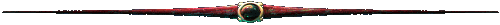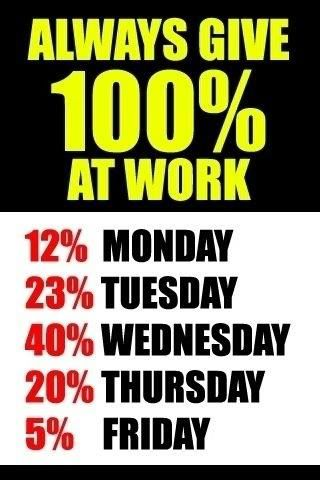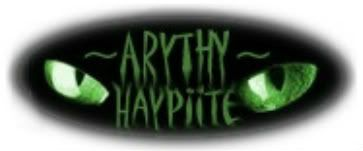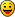## Movement formulaWarlord

Posts: 2282

Joined: Mon Apr 12, 2010 2:54 pm

### Movement formula

Sooooo Heres the movement formula in battle
Code:
`a= basic speedx= attribute (includes enchance/ equipmenty= Level of techa*(1+x/100+y*0,03)=movement`

Basis Speed:
Inf=150
Cav=300
arch=125
Cat=50

Example:

Cavalry basis speed = 300
max attribute=190
tech level = 10
300*(1+190/100+10*0,03)=
300*(1+1,9+0,3)=
300*3,2=
960
But you also have to consider that cav also got a range, the formula of range is
Code:
`b= basis rangec= tech lvlb*(1+c*0,02)=range`

Ranges:
Inf: 25
Cav: 40
Arch: 600
Cats: 600

so max movement of cats is 960 and max range is 40*(1+10*0,02)=48

maxed cav can reach enemies which are 960+48=1008 away.... that means
cav can hit now cats in the first round, if the speed of the opponent completes the equation: attribute/100+techlvl*3>=1,813333333 and the cats move forwardBRORANOSKing

Posts: 4618

Joined: Wed Oct 20, 2010 4:41 pm

### Re: Movement formula

thanks uranoshow do you let people know if your hot cakes are selling well?

Nathan05
S106Conqueror

Posts: 1331

Joined: Sun Jan 16, 2011 3:59 am

Location: Haypi Forums, Server 79 and Dragon S2 O.o

### Re: Movement formula

Thanks Uranos!I'll give any more guide suggestions as I think of them.

Also, I think max speed attribute is 167.5... (100 + 30 horse + 15 manual + 15 enhanced horse + 7.5 enhanced manual)
"If you're not confused, you're not paying attention."Haypi Support Emails:Veteran

Posts: 629

Joined: Wed May 12, 2010 6:34 pm

Location: At, or nowhere near my computer

### Re: Movement formula

Nice guide Uranos. One thing in it that confused me for a bit though...
uranos wrote:so max movement of cats is 960 and max range is 40*(1+10*0,02)=48
Beware the Artesians!!King

Posts: 4618

Joined: Wed Oct 20, 2010 4:41 pm

### Re: Movement formula

Arythy wrote:Thanks Uranos!I'll give any more guide suggestions as I think of them.

Also, I think max speed attribute is 167.5... (100 + 30 horse + 15 manual + 15 enhanced horse + 7.5 enhanced manual)

No max enhancement gives you 100% on equipment making the equipment = 45x2=90

Making max 100+90+30= 220
how do you let people know if your hot cakes are selling well?

Nathan05
S106Conqueror

Posts: 1331

Joined: Sun Jan 16, 2011 3:59 am

Location: Haypi Forums, Server 79 and Dragon S2 O.o

### Re: Movement formula

Ah, thanks Nathan. For some reason I recall the highest being 50%, I guess not."If you're not confused, you're not paying attention."Haypi Support Emails:King

Posts: 4618

Joined: Wed Oct 20, 2010 4:41 pm

### Re: Movement formula

no Enhancement adds 100% sso its nice round numbershow do you let people know if your hot cakes are selling well?

Nathan05
S106

Newbie

Posts: 24

Joined: Mon Jan 24, 2011 6:18 am

### Re: Movement formula

Nathan05 wrote:
Arythy wrote:Thanks Uranos!I'll give any more guide suggestions as I think of them.

Also, I think max speed attribute is 167.5... (100 + 30 horse + 15 manual + 15 enhanced horse + 7.5 enhanced manual)

No max enhancement gives you 100% on equipment making the equipment = 45x2=90

Making max 100+90+30= 220

What is the +30 from?
S44/111 - koarandyKing

Posts: 4618

Joined: Wed Oct 20, 2010 4:41 pm

### Re: Movement formula

+30 from the tech addded onto the base attribute
how do you let people know if your hot cakes are selling well?

Nathan05
S106

Newbie

Posts: 26

Joined: Wed Apr 20, 2011 1:14 am

### Re: Movement formula

Almost works, except that the remainders of a*(1 + x/100) and a*(y*0.03) are rounded down or up perhaps individually so the end result can be off by a unit or two. For instance, if a=50, x= 99, y = 9

then your equation would give you a distance traveled of

50*(1+99/100+9*.03) = 50*(2.26) = 113 when the actual movement is 112

although...

50*2.26 = 50 + 50*.99 + 50*.27 = 50 + 49.5 + 13.5 = 113
if you round down 49.5 and 13.5 to 49 and 13 the sum becomes 112 which is the correct speed, so i originally thought decimals were rounded down individually...

However,

125*2.26 = 282.5 = 125 + 123.75 + 33.75 = 282.5 and 125 + 123 +33 = 281 and 125 + 124 + 34 = 283 so neither rounding down nor up individually accounts for the error and neither rounding up nor down to the whole accounts for the error so I'm not exactly sure how its calculated.
Next